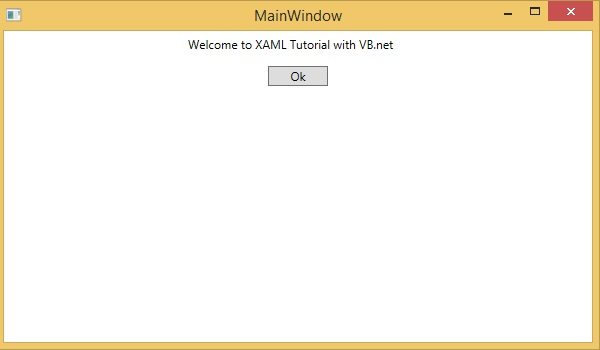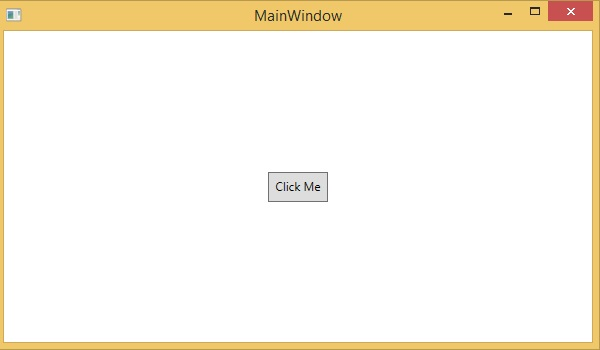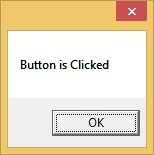# XAML Vs. VB.NET

In this chapter, we will write the same example in VB.Net so that those who are familiar with VB.Net can also understand the advantages of XAML.

Let’s take a look at the the same example again which is written in XAML −

```<Window x:Class = "XAMLVsCode.MainWindow"
xmlns = "http://schemas.microsoft.com/winfx/2006/xaml/presentation"
xmlns:x = "http://schemas.microsoft.com/winfx/2006/xaml" Title = "MainWindow" Height = "350" Width = "604">

<StackPanel>
<TextBlock Text = "Welcome to XAML Tutorial with VB.net" Height = "20" Width = "220" Margin = "5"/>
<Button Content = "Ok" Height = "20" Width = "60" Margin = "5"/>
</StackPanel>

</Window>
```

In this example, we have created a stack panel with a button and a Text block and defined some of the properties of the button and the text block such as Height, Width, and Margin. When the above code is compiled and executed, it will produce the following output −Now look at the same code which is written in VB.Net −

```Public Class MainWindow
Private Sub Window_Loaded(sender As Object, e As RoutedEventArgs)
Dim panel As New StackPanel()
panel.Orientation = Orientation.Vertical
Me.Content = panel
Dim txtInput As New TextBlock

txtInput.Text = "Welcome to XAML Tutorial with VB.net"
txtInput.Width = 220
txtInput.Height = 20
txtInput.Margin = New Thickness(5)

Dim btn As New Button()

btn.Content = "Ok"
btn.Width = 60
btn.Height = 20
btn.Margin = New Thickness(5)

End Sub
End Class
```

When the above code is compiled and executed the output is exactly the same as the output of XAML code.You can now visualize how simple it is to work with XAML as compared to VB.Net.

In the above example, we have seen that what we can do in XAML can also be done in other procedural languages such as C# and VB.Net.

Let’s have a look at another example in which we will use both XAML and VB.Net. We will design a GUI in XAML and the behavior will be implemented in VB.Net.

In this example, a button is added to the main window. When the user clicks this button, it displays a message on the message box. Here is the code in XAML in which a Button Object is declared with some properties.

```<Window x:Class="MainWindow"
xmlns = "http://schemas.microsoft.com/winfx/2006/xaml/presentation"
xmlns:x = "http://schemas.microsoft.com/winfx/2006/xaml" Title = "MainWindow" Height = "350" Width = "604">

<Grid>
<Button Name = "btn" HorizontalAlignment = "Center" Width = "60" Height = "30" Content = "Click Me" />
</Grid>

</Window>
```

In VB.Net, the button click event (behavior) is implemented. This event displays the message on the messagebox.

```Public Class MainWindow
Private Sub btn_Click(sender As Object, e As RoutedEventArgs) Handles btn.Click
MessageBox.Show("Button is Clicked")
End Sub
End Class
```

When the above code is compiled and executed, it will display the following screen −Now click on the above button that says "Click Me". It will display the following message −